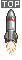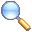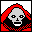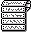Jack And The Beanstalk
Up The Beanstalk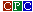Last Update : Saturday 09 October 2021 at 16 h 02CTM644 Colour MonitorGT65 Green Monitor

## INFOS

- CATEGORIES -
- NUMBER OF PLAYERS -
1 player
- DESCRIPTION -

My name is Jack and I live with my mother in a small house. We are very poor and my mother is always telling me off for being lazy. 'Get a job', she says, but with the high rate of unemployment, that has proved to be very difficult.

I have heard of an evil Ogre who lives not far from here and who is reputed to have a goose that lays golden eggs, a large bag of money and a golden harp which he stole from the peasants many years ago. I have made up my mind to try and recover these treasures and take them to my mother - but I can only carry one item at a time.

- CONTROLS -

* Liste des commandes acceptées par le jeu :
N, S, W, E, IN, OUT, UP, DOWN, QUIT, SCORE, READ, SOW, PLANT, WEAR, REMOVE, EAT, DRINK, HELP, KILL, STAB, ATTACK, PULL, RUB, SWIM, UNLOCK, INVENTORY, GET, TAKE, GRAB, DROP, LEAVE, PUT, CLIMB, HIDE, GIVE, CHOP, CUT, SEARCH, OPEN, WAIT

Enregistrer la partie : SAVE
Charger une partie sauvegardée : LOAD

- STAFF -

Paru sous forme de listing dans le magazine Amstrad Computer User (Jan/Feb 1985).
Auteur : Steve W. LUCAS

- BUGS -

a) Le message "The Ogre catches me and throws me to the snakes!" n'apparaît pas lorsque le personnage se cache deux fois dans le placard
AVANT :
1680 IF ae=1 AND z\$="cup" THEN PRINT x\$(1):ae=2:p%=64:s%=s%+5:ax=1:RETURN ELSE IF z\$=n\$(14) THEN x\$="The Ogre catches me and throws me to thesnakes!":GOSUB 1290

APRES :
1680 IF ae=1 AND z\$="cup" THEN PRINT x\$(1):ae=2:p%=64:s%=s%+5:ax=1:RETURN ELSE IF z\$="cup" THEN x\$="The Ogre catches me and throws me to thesnakes!":GOSUB 1290

b) Le message "'FEE FIE FOE FUM' says the Ogre as he grabs me from behind and casts me to the rats" n'apparaît pas lorsque le personnage se cache deux fois dans la lessiveuse
AVANT :
1700 IF ae=2 AND z\$="cop" THEN PRINT x\$(1):p%=67:ae=3:s%=s%+5:RETURN ELSE IF z\$=n\$(13) THEN x\$="'FEE FIE FOE FUM' says the Ogre as he grabs me from behind and casts me to therats":GOSUB 1290

APRES :
1700 IF ae=2 AND z\$="cop" THEN PRINT x\$(1):p%=67:ae=3:s%=s%+5:RETURN ELSE IF z\$="cop" THEN x\$="'FEE FIE FOE FUM' says the Ogre as he grabs me from behind and casts me to therats":GOSUB 1290

c) Le personnage reste anormalement déguisé après s'être débarrassé de son déguisement via la commande DROP
AVANT :
1170 b%(n%(r))=p%:IF r=2 THEN ah=0 ELSE IF r=6 AND p%=24 THEN GOSUB 1830

APRES :
1170 b%(n%(r))=p%:IF r=2 THEN ah=0 ELSE IF r=6 AND p%=24 THEN GOSUB 1830 ELSE IF (r=11 OR r=12) AND ai=1 THEN ai=0 ELSE IF r=20 AND aj=1 THEN aj=0

d) La commande LOAD ne permet pas de restaurer une partie sauvegardée
AVANT :
1420 FOR x=1 TO 78:PRINT#9,q\$(x):NEXT x

APRES :
1420 FOR x=1 TO 78:WRITE#9,q\$(x):NEXT x

AVANT :
1430 FOR x=1 TO 78:FOR y=1 TO 4:PRINT#9,s%(x,y):NEXT y,x

APRES :
1430 FOR x=1 TO 78:FOR y=1 TO 4:WRITE#9,s%(x,y):NEXT y,x

AVANT :
1440 FOR x=1 TO 23:PRINT#9,g\$(x):NEXT x:FOR x=1 TO 23:PRINT#9,b%(x):NEXT x:FOR x=1 TO 27:PRINT#9,n\$(x):NEXT x:FOR x=1 TO 27:PRINT#9,n%(x):NEXT x

APRES :
1440 FOR x=1 TO 23:WRITE#9,g\$(x):NEXT x:FOR x=1 TO 23:WRITE#9,b%(x):NEXT x:FOR x=1 TO 27:WRITE#9,n\$(x):NEXT x:FOR x=1 TO 27:WRITE#9,n%(x):NEXT x

AVANT :
1450 FOR x=1 TO 34:PRINT#9,x\$(x):NEXT x:FOR x=1 TO 4:PRINT#9,v\$(x):NEXT x

APRES :
1450 FOR x=1 TO 34:WRITE#9,x\$(x):NEXT x:FOR x=1 TO 4:WRITE#9,v\$(x):NEXT x

AVANT :

APRES :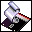ACU Anthology Volume 1 © Amstrad Computer User (1986)
Version Commodore 64 : "Lemon64"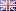Version Spectrum : "Spectrum Computing"